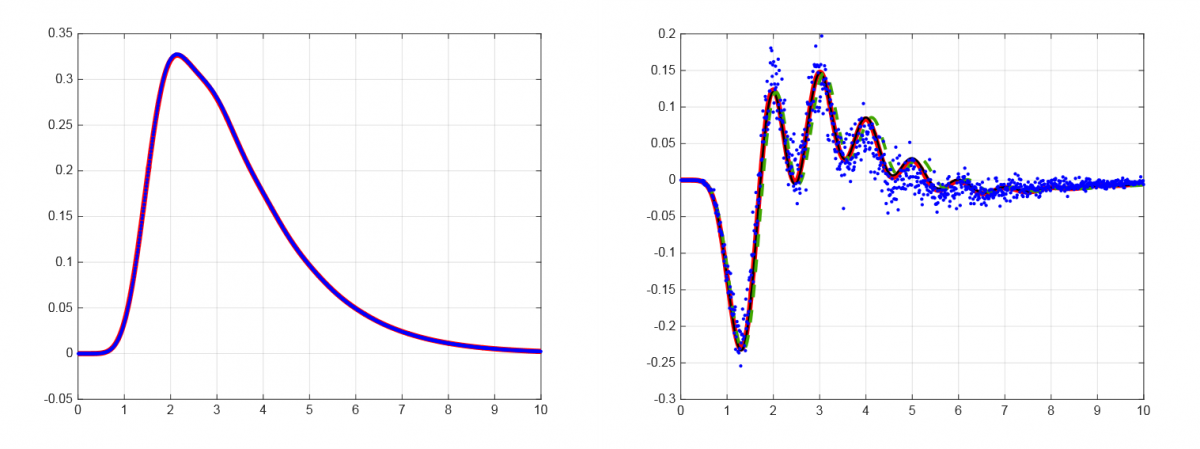# Random matrices and big data sets

From exact data, numerical extrapolation can be used to isolate finite size effects. In noisy data, only big data sets have small enough variances that such extrapolation is feasible. The Riemann zeros from the theory of prime numbers provide an example of an exact big data set which paradoxically behave like noising data. Although over 10^9 consecutive Riemann zeros beginning beyond zero number 10^{23} have been computed to high accuracy, finite size effects are on the scale of the logarithm of this number. Studies completed on this data set in 2015 (Forrester and Mays) and 2016 (Bornemann, Forrester and Mays) have demonstrated the validity of an hypothesis first put forward by Keating and Snaith relating the leading order finite size correction to random matrix theory. Two lines of future work suggest themselves: how to systematically compute large N expansions in random matrix theory, and the investigation of the theoretical mechanism behind the correction term for the Riemann zero statistics.## fig2.png

Left caption: leading order spacing statistic for the Riemann zeros with 40% thinning (blue), random matrix prediction red curve.

Right caption: the subleading correction for the same Riemann zeros statistic (blue dots), plotted again the random matrix prediction.